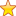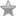# How to Find out the LCM of Three Numbers – C Program

## How to Find LCM of three numbers

Please write your version or code in other Languages below in comments.

You will find code in these languages:-

• C
• C++
• Java

If you can write a program to find LCM of two numbers. Then you can very easily write a program to find LCM of three numbers also , cause lcm(a,b,c)=lcm(a,lcm(b,c))lcm(a,b,c)=lcm(a,lcm(b,c)).

So here’s your c program to find LCM of three numbers.

### C Program to find lcm of 3 numbers

[code language=”cpp”]
#include<stdio.h>

int lcm(int,int);
int main(){
int a,b,c,l,k;
printf("Enter any three positive integers ");
scanf("%d%d%d",&a,&b,&c);
if(a<b)
l = lcm(a,b);
else

l = lcm(b,a);

if(l>c)

k= lcm(l,c);

else

k= lcm(c,l);

printf("LCM of two integers is %d",k);

return 0;

}

int lcm(int a,int b){

int temp = a;

while(1){

if(temp % b == 0 && temp % a == 0)

break;

temp++;

}

return temp;
}

[/code]

### Code in C++

[code language=”cpp”]

#include<iostream>
using namespace std;

int lcm(int, int, int);
int hcf(int, int, int);
int main()
{
int a,b,c;
int LCM, HCF;
cout<<"Enter 1st number: ";
cin>>a;
cout<<"Enter 2nd number: ";
cin>>b;
cout<<"Enter 3rd number: ";
cin>>c;

LCM = lcm(a,b,c);
HCF = hcf(a,b,c);
cout<<"LCM of "<<a<<","<<b<<","<<c<<" is = "<<LCM<<endl;
cout<<"HCF of "<<a<<","<<b<<","<<c<<" is = "<<HCF<<endl;
return 0;
}
int lcm(int x,int y, int z)
{
long max,lcom, count, flag=0;
if(x>=y&&x>=z)
max=x;
else if(y>=x&&y>=z)
max=y;
else if(z>=x&&z>=y)
max=z;
for(count=1;flag==0;count++)
{
lcom=max*count;
if(lcom%x==0 && lcom%y==0 && lcom%z==0)
{
flag=1;
}
}
return lcom;
}

int hcf(int p, int q, int r)
{
int gcf=1,flag=0, count;
for(count=1; flag==0;count++)
{
if(p%count==0&&q%count==0&&r%count==0)
gcf=count;
if(count>p&&count>q&&count>r)
{
flag=1;
}
}
return gcf;
}
[/code]

### Java Program to Find LCM of N Numbers

LCM of three Numbers in Java

[code language=”java”]
public class LCM {

public static long lcm(int[] numbers) {
long lcm = 1;
int divisor = 2;
while (true) {
int cnt = 0;
boolean divisible = false;
for (int i = 0; i < numbers.length; i++) {
/**
* lcm (n1,n2,… 0)=0.For negative number we convert into
* positive and calculate lcm.
*/
if (numbers[i] == 0) {
return 0;
} else if (numbers[i] < 0) {
numbers[i] = numbers[i] * (-1);
}
if (numbers[i] == 1) {
cnt++;
}
/**
* divide numbers by devisor if complete division i.e. without
* remainder then replace number with quotient; used for find
* next factor
*/
if (numbers[i] % divisor == 0) {
divisible = true;
numbers[i] = numbers[i] / divisor;
}
}
/**
* If divisor able to completely divide any number from array
* multiply with lcm and store into lcm and continue to same divisor
* for next factor finding. else increment divisor
*/
if (divisible) {
lcm = lcm * divisor;
} else {
divisor++;
}
/**
* Check if all numbers is 1 indicate we found all factors and
* terminate while loop.
*/
if (cnt == numbers.length) {
return lcm;
}
}
}

public static int lcm2(int num1, int num2) {
if(num1==0 || num2==0){
return 0;
}else if(num1<0){
num1=num1*(-1);
}else if(num2<0){
num2=num2*(-1);
}
int m = num1;
int n = num2;
while (num1 != num2) {
if (num1 < num2) {
num1 = num1 + m;
} else {
num2 = num2 + n;
}
}
return num1;
}

public static void main(String[] args) {
int[] numbers = {140, 72, 130};
System.out.println("*** Least Common Multiple ***");
System.out.println("LCM(Least Common Multiple) of N numbers using Table method ");
System.out.println(lcm(numbers));
System.out.println("LCM of two numbers using repetative addition");
System.out.println(lcm2(1, 72));

}
}
[/code]

#### Output:

*** Least Common Multiple ***
LCM(Least Common Multiple) of N numbers using Table method
32760
LCM of two numbers using repetative addition
72

Summary
Review Date
Reviewed Item
LCM of three numbers
Author Rating
4